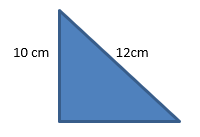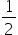Maths-
General
Easy

Question

# Find the area of a given triangle whose perimeter is equal to 26cm## The correct answer is: 20 sq cm

### Given:Perimeter = 26cmHeight = 10cmHypotenuse = 12cmArea = ?Perimeter of triangle = sum of sides26 = 10 + 12 + b26 = 22 + b26 - 22 = b4cm = bArea =× base × height=× 4 × 10= 20 sq. cm#### With Turito Foundation.#### Get an Expert Advice From Turito.